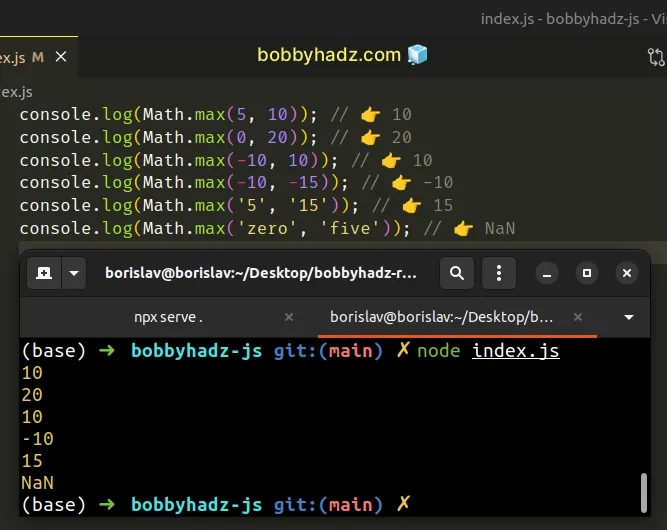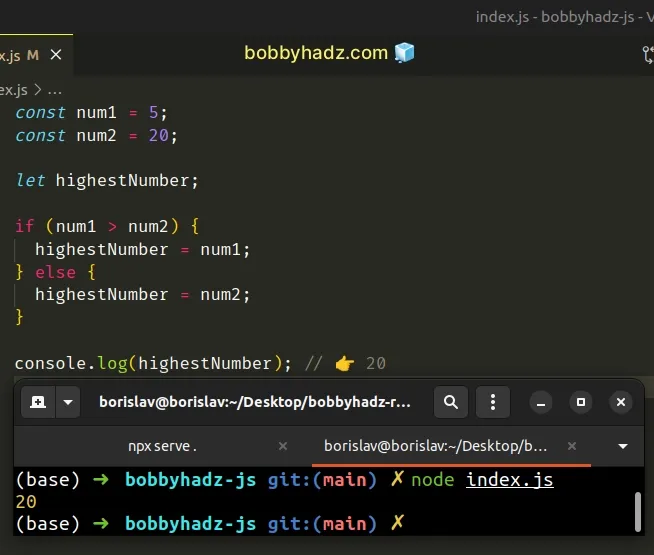# Get the Max of two Numbers using JavaScriptLast updated: Jan 7, 2023
3 min## #Get the Max of two Numbers in JavaScript

Use the `Math.max()` function to get the max of 2 numbers, e.g. `Math.max(10, 5)`.

The function returns the largest of the provided numbers. If any of the provided parameters is not a number and can't be converted to one, `NaN` is returned.

index.js
```Copied!```console.log(Math.max(5, 10)); // 👉️ 10
console.log(Math.max(0, 20)); // 👉️ 20
console.log(Math.max(-10, 10)); // 👉️ 10
console.log(Math.max(-10, -15)); // 👉️ -10
console.log(Math.max('5', '15')); // 👉️ 15
console.log(Math.max('zero', 'five')); // 👉️ NaN
``````We used the Math.max() function to get the max of two numbers.

The function takes zero or more numbers as parameters and returns the largest number.

Therefore you could provide more than 2 numbers to the function.

index.js
```Copied!```console.log(Math.max(1, 3, 5, 7)); // 👉️ 7
``````

If any of the provided values are not of type number, the function attempts to convert the value to a number before making the comparison.

If the function fails to convert the value to a number, it returns `NaN` (not a number).

## #Get the Max of two numbers using the ternary operator

You can also get the max of two numbers by using the ternary operator.

index.js
```Copied!```const num1 = 5;
const num2 = 20;

const highestNumber = num1 > num2 ? num1 : num2;

console.log(highestNumber); // 👉️ 20
``````The ternary operator is very similar to an `if/else` statement.

If the expression to the left of the question mark is truthy, the operator returns the value to the left of the colon, otherwise, the value to the right of the colon is returned.

If `num1` is greater than `num2`, then `num1` is returned, otherwise, `num2` is returned.

## #Get the Max of two numbers using an `if/else` statement

You can also use an `if/else` statement.

index.js
```Copied!```const num1 = 5;
const num2 = 20;

let highestNumber;

if (num1 > num2) {
highestNumber = num1;
} else {
highestNumber = num2;
}

console.log(highestNumber); // 👉️ 20
``````Notice that we used the `let` keyword to declare the `highestNumber` variable.

Variables declared using `const` cannot be reassigned.

In the `if` statement we check if `num1` is greater than `num2` and if the condition is met, we set the `highestNumber` variable to `num1`.

Otherwise, we set the variable to `num2`.

## #If you have the numbers stored in an array, use spread syntax (...)

The `Math.max()` function takes multiple, comma-separated numbers, so we can't directly pass it an array of numbers.

index.js
```Copied!```const numbers = [5, 0, 20, 13, 35];

const result = Math.max(...numbers);
console.log(result); // 👉️ 35
``````We used the spread syntax (...) to unpack the array in the call to the `Math.max()` function.

You can imagine that the function got called with multiply, comma-separated arguments with the help of the spread syntax (...).

## #Some non-numeric values convert to valid numbers

If the `Math.max()` function gets called with a value that is not a number, the function attempts to convert the value to a number before making the comparison.

Note that some non-numeric values get converted to valid numbers in JavaScript. Here are some examples.

index.js
```Copied!```console.log(Number([])); // 👉️ 0
console.log(Number(null)); // 👉️ 0
console.log(Number(false)); // 👉️ 0
console.log(Number(true)); // 👉️ 1
console.log(Number('')); // 👉️ 0
``````

If you pass any of these values to the `Math.max()` function, you might get confusing results.

index.js
```Copied!```console.log(Math.max(null, -10)); // 👉️ 0
console.log(Math.max([], -10)); // 👉️ 0
console.log(Math.max(true, -10)); // 👉️ 1
``````

In these examples, the `Math.max` function converted the first value to a number before comparing the values.

Since all of these successfully convert to a number, we got some unexpected results.